In this tutorial we demonstrate the adaptive solution of free surface problems on unstructured meshes, using the example of a bubble propagating along a straight channel. We also demonstrate how to impose volume constraints on enclosed regions within the fluid.

# The example problem

We illustrate the solution of the unsteady 2D Navier-Stokes equations by considering the propagation of a single bubble along a straight channel as shown in the sketch below. We non-dimensionalise all lengths on the channel width,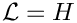, velocities on the maximum (prescribed) inflow velocity,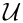, and time on the intrinsic timescale,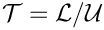, which corresponds to a Strouhal number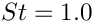.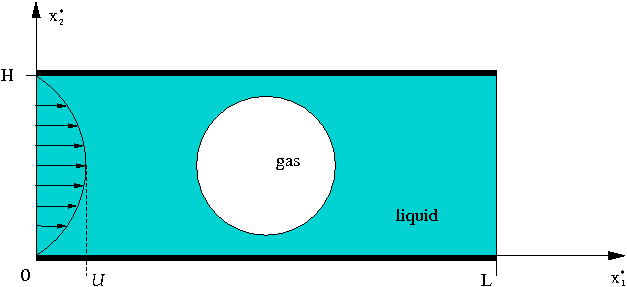The problem setup.

The problem is then governed by the following equations.

Solve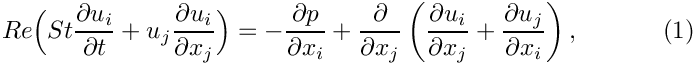and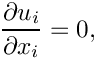in the rectangular domain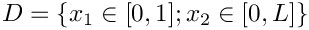, subject to the Dirichlet boundary conditions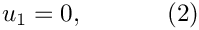on the top and bottom boundaries and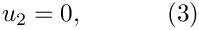on all boundaries. The inflow on the left boundary is described by a Poiseuille flow profile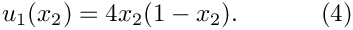The free surface is defined by the position vector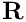, which is subject to the kinematic condition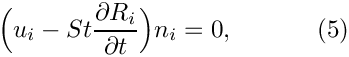and the dynamic condition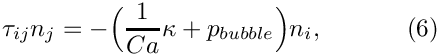where the stress tensor is defined as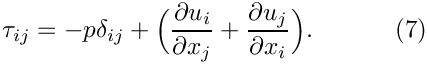The initial position of the interface, for a bubble initially located in the centre of the channel, is given by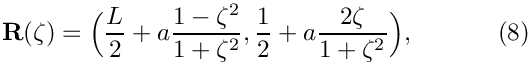where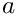is the initial non-dimensional bubble radius and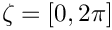.

The problem is subject to the constraint that the bubble volume,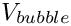, remains constant which is achieved by adjusting, the bubble pressure,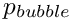. We formulate the volume constraint by making use of Gauss theorem, which states that for any vector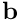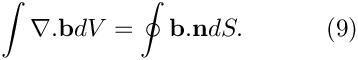Choosing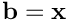we note that the divergence of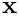gives the spatial dimension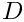and the integral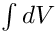is the enclosed volume. Hence, the volume constraint can be written as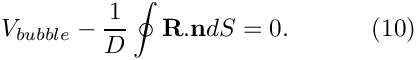This is the equation that determines the unknown bubble pressure.

# Implementation

We solve the governing equations using an ALE-based finite-element method, discretising the fluid domain with triangular Taylor-Hood elements, and updating the mesh with a pseudo-elastic node-update procedure.
As usual, we impose the kinematic and dynamic boundary conditions with FaceElements. The volume constraint is imposed in a similar way: We attach LineVolumeConstraintBoundingSolidElements to the bubble surface to compute the line integrals in equation (10), and create an additional VolumeConstraintElement which addsto this equation and is also "in charge" of the unknown bubble pressure.

# Results

We perform the simulation in a two-stage procedure. We start by performing a steady solve with the inflow switched off. This deforms the bubble into its steady state (approximately) circular configuration with the required volume. The actual time-dependent simulation is then performed with an impulsive start from this configuration.

The figure below shows a contour plot of the pressure distribution with overlaid streamlines. This is a snapshot of an animation of the flow field, for the parameters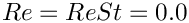and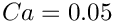.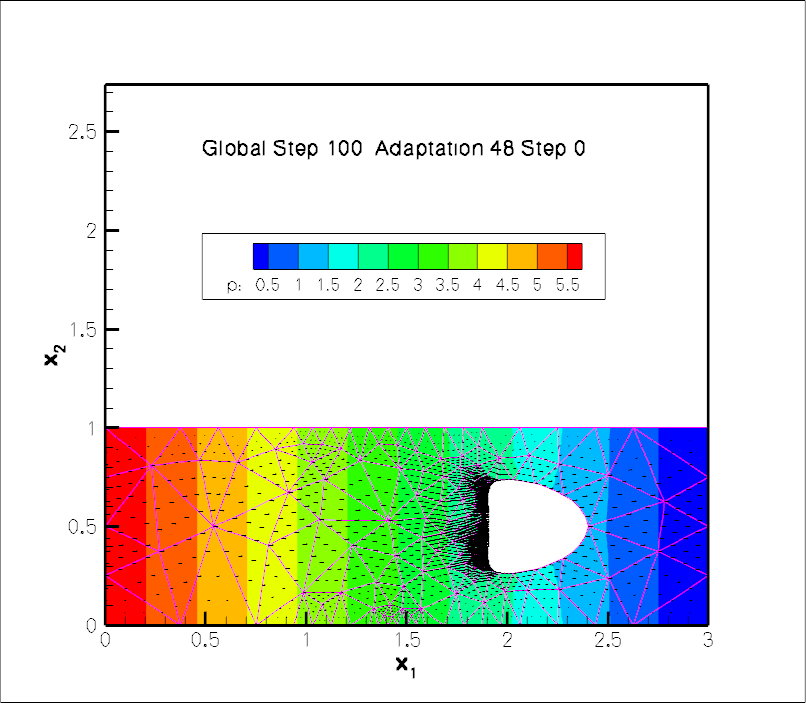Snapshot of the flow field (streamlines and pressure contours) for a propagating bubble.

# Global parameters

As usual, we create a namespace where we define the dimensionless parameters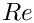,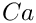and the non-dimensional channel length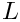. As discussed in another tutorial, the Strouhal number defaults to one, so that we do not have to set it in this case. We also store the initial bubble radius and the bubble volume, as well as a scaling factor for the inflow velocity (this allows us to "switch off" the inflow when computing the initial steady solution). Finally we define the Poisson ratio for the generalised Hookean constitutive law that is used by pseudo-elastic mesh update.

//==start_of_namespace==============================
/// Namespace for Problem Parameter
//==================================================
{
/// Doc info object
DocInfo Doc_info;
/// Reynolds number
double Re=0.0;
/// Capillary number
double Ca = 10.0;
/// Pseudo-solid Poisson ratio
double Nu=0.3;
/// Volume of the bubble (negative because it's outside the
/// fluid!)
/// Scaling factor for inflow velocity (allows it to be switched off
/// to do hydrostatics)
double Inflow_veloc_magnitude = 0.0;
/// Length of the channel
double Length = 3.0;
/// Constitutive law used to determine the mesh deformation
ConstitutiveLaw *Constitutive_law_pt=0;
/// Trace file
ofstream Trace_file;
/// File to document the norm of the solution (for validation
/// purposes -- triangle doesn't give fully reproducible results so
/// mesh generation/adaptation may generate slightly different numbers
/// of elements on different machines!)
ofstream Norm_file;
} // end_of_namespace
////////////////////////////////////////////////////////////// //////////////////////////////////////...
DocInfo Doc_info
Doc info object.
ofstream Norm_file
File to document the norm of the solution (for validation purposes – triangle doesn't give fully repr...
ofstream Trace_file
Trace file.
double Inflow_veloc_magnitude
Scaling factor for inflow velocity (allows it to be switched off to do hydrostatics)
double Length
Length of the channel.
ConstitutiveLaw * Constitutive_law_pt
Constitutive law used to determine the mesh deformation.
double Volume
Volume of the bubble (negative because it's outside the fluid!)
double Nu
Pseudo-solid Poisson ratio.
double Re
Reynolds number.
double Ca
Capillary number.

# The driver code

We start by processing the command line arguments and create the generalised Hookean constitutive equations for the pseudo-elastic node-update. We then open various output files and build the problem.

//==========start_of_main=====================================
/// Driver code for moving bubble problem
//============================================================
int main(int argc, char **argv)
{
// Store command line arguments
CommandLineArgs::setup(argc,argv);
// Define possible command line arguments and parse the ones that
// were actually specified
// Validation?
CommandLineArgs::specify_command_line_flag("--validation");
// Parse command line
CommandLineArgs::parse_and_assign();
// Doc what has actually been specified on the command line
CommandLineArgs::doc_specified_flags();
// Create generalised Hookean constitutive equations
new GeneralisedHookean(&Problem_Parameter::Nu);
// Open trace file
Problem_Parameter::Trace_file.open("RESLT/trace.dat");
// Increase precision of output
// Open norm file
Problem_Parameter::Norm_file.open("RESLT/norm.dat");
// Create problem in initial configuration
int main(int argc, char **argv)
Driver code for moving bubble problem.
//////////////////////////////////////////////////////// ////////////////////////////////////////////...

We start by performing a steady solve (with the inflow switched off) to compute the initial configuration, a circular bubble in stationary fluid.

// Before starting the time-integration we want to "inflate" it to form
// a proper circular bubble. We do this by setting the inflow to zero
// If all went well, this should show us a nice circular bubble
// in a stationary fluid
problem.doc_solution();

Next, the timestepper is initialised and an impulsive start performed. The inflow is switched on and the first few unsteady Newton solves are performed without adaptation.

// Initialise timestepper
double dt=0.025;
problem.initialise_dt(dt);
// Perform impulsive start from current state
problem.assign_initial_values_impulsive();
// Now switch on the inflow and re-assign the boundary conditions
// (Call to complete_problem_setup() is a bit expensive given that we
// we only want to set the inflow velocity but who cares -- it's just
// a one off.
problem.complete_problem_setup();
// Solve problem on fixed mesh
unsigned nstep=6;
if (CommandLineArgs::command_line_flag_has_been_set("--validation"))
{
nstep=2;
oomph_info << "Remeshing after every second step during validation\n";
}
for (unsigned i=0;i<nstep;i++)
{
// Solve the problem
problem.doc_solution();
} // done solution on fixed mesh

To limit the distortion of the elements we allow then mesh adaptation (which involves the re-generation of the entire mesh) every few timesteps.

// Now do a proper loop, doing nstep timesteps before adapting/remeshing
// and repeating the lot ncycle times
unsigned ncycle=1000;
if (CommandLineArgs::command_line_flag_has_been_set("--validation"))
{
ncycle=1;
oomph_info << "Only doing one cycle during validation\n";
}
// Do the cycles
for(unsigned j=0;j<ncycle;j++)
{
// Allow up to one level of refinement for next solve
//Solve problem a few times
for (unsigned i=0;i<nstep;i++)
{
// Solve the problem
// Build the label for doc and output solution
std::stringstream label;
label << "Adaptation " <<j << " Step "<< i;
problem.doc_solution(label.str());
// No more refinement for the next nstep steps
}
}
} //End of main

# The problem class

As usual, we template the Problem class by the element type

//==start_of_problem_class============================================
/// Problem class to simulate inviscid bubble propagating along 2D channel
//====================================================================
template<class ELEMENT>
class BubbleInChannelProblem : public Problem
{
public:
/// Constructor
/// Destructor

The FaceElements are deleted and re-attached before and after each adaptation. Also, as discussed in another tutorial, we re-apply the boundary conditions and complete the build of all elements after each adaptation, using the helper function complete_problem_setup() (discussed below).

/// Actions before adapt: Wipe the mesh of free surface elements
{
// Kill the elements and wipe surface mesh
delete_free_surface_elements();
delete_volume_constraint_elements();
// Rebuild the Problem's global mesh from its various sub-meshes
this->rebuild_global_mesh();
/// Actions after adapt: Rebuild the mesh of free surface elements
{
// Create the elements that impose the displacement constraint
create_free_surface_elements();
create_volume_constraint_elements();
// Rebuild the Problem's global mesh from its various sub-meshes
this->rebuild_global_mesh();
// Setup the problem again -- remember that fluid mesh has been
// completely rebuilt and its element's don't have any
// pointers to Re etc. yet
complete_problem_setup();
/// Update the after solve (empty)
void actions_after_newton_solve(){}
/// Update the problem specs before solve
void actions_before_newton_solve()
{
//Reset the Lagrangian coordinates of the nodes to be the current
//Eulerian coordinates -- this makes the current configuration
//stress free
Fluid_mesh_pt->set_lagrangian_nodal_coordinates();
}
/// Set boundary conditions and complete the build of all elements
void complete_problem_setup();

We define the post-processing functions to document the solution and to compute the error estimates.

/// Doc the solution
void doc_solution(const std::string& comment="");
/// Compute the error estimates and assign to elements for plotting
void compute_error_estimate(double& max_err,

We also provide helper functions to delete and create face elements adjacent to the bubble boundary.

private:
/// Create free surface elements
void create_free_surface_elements();
/// Delete free surface elements
void delete_free_surface_elements()
{
// How many surface elements are in the surface mesh
unsigned n_element = Free_surface_mesh_pt->nelement();
// Loop over the surface elements
for(unsigned e=0;e<n_element;e++)
{
// Kill surface element
delete Free_surface_mesh_pt->element_pt(e);
}
// Wipe the mesh
Free_surface_mesh_pt->flush_element_and_node_storage();
} // end of delete_free_surface_elements
/// Create elements that impose volume constraint on the bubble
void create_volume_constraint_elements();
/// Delete volume constraint elements
void delete_volume_constraint_elements()
{
// How many surface elements are in the surface mesh
unsigned n_element = Volume_constraint_mesh_pt->nelement();
// Loop over the surface elements (but don't kill the volume constraint
// element (element 0))
unsigned first_el_to_be_killed=1;
for(unsigned e=first_el_to_be_killed;e<n_element;e++)
{
delete Volume_constraint_mesh_pt->element_pt(e);
}
// Wipe the mesh
Volume_constraint_mesh_pt->flush_element_and_node_storage();
} // end of delete_volume_constraint_elements

The private data includes pointers to the fluid mesh, and the two face meshes which impose the kinematic and dynamic boundary conditions, and the volume constraint, respectively. We also store pointers to the Data that stores the unknown bubble pressure and to the VolumeConstraintElement that imposes the volume constraint and is "in charge of" the bubble pressure.

/// Pointers to mesh of free surface elements
Mesh* Free_surface_mesh_pt;
/// Pointer to mesh containing elements that impose volume constraint
Mesh* Volume_constraint_mesh_pt;
/// Pointer to Fluid_mesh
RefineableSolidTriangleMesh<ELEMENT>* Fluid_mesh_pt;
/// Vector storing pointer to the bubble polygons
Vector<TriangleMeshPolygon*> Bubble_polygon_pt;
/// Triangle mesh polygon for outer boundary
TriangleMeshPolygon* Outer_boundary_polyline_pt;
/// Pointer to a global bubble pressure datum
Data* Bubble_pressure_data_pt;
/// Pointer to element that imposes volume constraint for bubble
VolumeConstraintElement* Vol_constraint_el_pt;
/// Enumeration of mesh boundaries
enum
{
Inflow_boundary_id=0,
Upper_wall_boundary_id=1,
Outflow_boundary_id=2,
Bottom_wall_boundary_id=3,
First_bubble_boundary_id=4,
Second_bubble_boundary_id=5
};
}; // end_of_problem_class

# The problem constructor

We allocate the timestepper and build the VolumeConstraintElement that imposes the volume constraint. The element stores the volume that is to be conserved as well as the bubble pressure, which is determined from the volume constraint. The initial guess for the bubble pressure,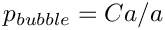, is appropriate for a static bubble in stationary fluid.

//==start_constructor=====================================================
/// Constructor
//========================================================================
template<class ELEMENT>
{
// Output directory
Problem_Parameter::Doc_info.set_directory("RESLT");
// Allocate the timestepper -- this constructs the Problem's
// time object with a sufficient amount of storage to store the
// previous timsteps.
// Build volume constraint element: Pass pointer to double that
// specifies target volume, data that contains the "traded" pressure
// and the index of the traded pressure value within this Data item
// Build element and create pressure internally
Vol_constraint_el_pt=
new VolumeConstraintElement(&Problem_Parameter::Volume);
// Which value stores the pressure?
// Pressure data

Next the outer boundary, consisting of four separate polylines, is built. Each polyline is defined by a start and an end point and is stored in a vector. This vector is then used to create the closed polygon required by Triangle.

// Build the boundary segments for outer boundary, consisting of
//--------------------------------------------------------------
// four separate polylines
//------------------------
Vector<TriangleMeshCurveSection*> boundary_polyline_pt(4);
// Each polyline only has two vertices -- provide storage for their
// coordinates
Vector<Vector<double> > vertex_coord(2);
for(unsigned i=0;i<2;i++)
{
vertex_coord[i].resize(2);
}
// First polyline: Inflow
vertex_coord=0.0;
vertex_coord=0.0;
vertex_coord=0.0;
vertex_coord=1.0;
// Build the 1st boundary polyline
boundary_polyline_pt = new TriangleMeshPolyLine(vertex_coord,
Inflow_boundary_id);
// Second boundary polyline: Upper wall
vertex_coord=0.0;
vertex_coord=1.0;
vertex_coord=Problem_Parameter::Length;
vertex_coord=1.0;
// Build the 2nd boundary polyline
boundary_polyline_pt = new TriangleMeshPolyLine(vertex_coord,
Upper_wall_boundary_id);
// Third boundary polyline: Outflow
vertex_coord=Problem_Parameter::Length;
vertex_coord=1.0;
vertex_coord=Problem_Parameter::Length;
vertex_coord=0.0;
// Build the 3rd boundary polyline
boundary_polyline_pt = new TriangleMeshPolyLine(vertex_coord,
Outflow_boundary_id);
// Fourth boundary polyline: Bottom wall
vertex_coord=Problem_Parameter::Length;
vertex_coord=0.0;
vertex_coord=0.0;
vertex_coord=0.0;
// Build the 4th boundary polyline
boundary_polyline_pt = new TriangleMeshPolyLine(vertex_coord,
Bottom_wall_boundary_id);
// Create the triangle mesh polygon for outer boundary
Outer_boundary_polyline_pt = new TriangleMeshPolygon(boundary_polyline_pt);

Next the polygon representing the bubble in the initial setup is generated. (Recall that closed polygons must be subdivided into at least two distinct polylines.)

// Now define initial shape of bubble(s) with polygon
//---------------------------------------------------
// We have one bubble
Bubble_polygon_pt.resize(1);
// Place it smack in the middle of the channel
double x_center = 0.5*Problem_Parameter::Length;
double y_center = 0.5;
Ellipse * bubble_pt = new Ellipse(Problem_Parameter::Radius,
// Intrinsic coordinate along GeomObject defining the bubble
Vector<double> zeta(1);
// Position vector to GeomObject defining the bubble
Vector<double> coord(2);
// Number of points defining bubble
unsigned npoints = 16;
double unit_zeta = MathematicalConstants::Pi/double(npoints-1);
// This bubble is bounded by two distinct boundaries, each
// represented by its own polyline
Vector<TriangleMeshCurveSection*> bubble_polyline_pt(2);
// Vertex coordinates
Vector<Vector<double> > bubble_vertex(npoints);
// Create points on boundary
for(unsigned ipoint=0; ipoint<npoints;ipoint++)
{
// Resize the vector
bubble_vertex[ipoint].resize(2);
// Get the coordinates
zeta=unit_zeta*double(ipoint);
bubble_pt->position(zeta,coord);
// Shift
bubble_vertex[ipoint]=coord+x_center;
bubble_vertex[ipoint]=coord+y_center;
}
// Build the 1st bubble polyline
bubble_polyline_pt = new TriangleMeshPolyLine(bubble_vertex,
First_bubble_boundary_id);
// Second boundary of bubble
for(unsigned ipoint=0; ipoint<npoints;ipoint++)
{
// Resize the vector
bubble_vertex[ipoint].resize(2);
// Get the coordinates
zeta=(unit_zeta*double(ipoint))+MathematicalConstants::Pi;
bubble_pt->position(zeta,coord);
// Shift
bubble_vertex[ipoint]=coord+x_center;
bubble_vertex[ipoint]=coord+y_center;
}
// Build the 2nd bubble polyline
bubble_polyline_pt = new TriangleMeshPolyLine(bubble_vertex,
Second_bubble_boundary_id);
// Define coordinates of a point inside the bubble
Vector<double> bubble_center(2);
bubble_center=x_center;
bubble_center=y_center;
// Create closed polygon from two polylines
Bubble_polygon_pt = new TriangleMeshPolygon(
bubble_polyline_pt,
bubble_center);

Once the boundary representation in form of polygons is completed the mesh is generated using Triangle. We specify the error estimator and set targets for the spatial adaptivity, and output the initial mesh.

// Now build the mesh, based on the boundaries specified by
//---------------------------------------------------------
// polygons just created
//----------------------
// Convert to "closed curve" objects
TriangleMeshClosedCurve* outer_closed_curve_pt=Outer_boundary_polyline_pt;
unsigned nb=Bubble_polygon_pt.size();
Vector<TriangleMeshClosedCurve*> bubble_closed_curve_pt(nb);
for (unsigned i=0;i<nb;i++)
{
bubble_closed_curve_pt[i]=Bubble_polygon_pt[i];
}
// Target area for initial mesh
double uniform_element_area=0.2;
// Use the TriangleMeshParameters object for gathering all
// the necessary arguments for the TriangleMesh object
TriangleMeshParameters triangle_mesh_parameters(
outer_closed_curve_pt);
// Define the holes on the boundary
triangle_mesh_parameters.internal_closed_curve_pt() =
bubble_closed_curve_pt;
// Define the maximum element areas
triangle_mesh_parameters.element_area() =
uniform_element_area;
// Create the mesh
Fluid_mesh_pt =
new RefineableSolidTriangleMesh<ELEMENT>(
triangle_mesh_parameters, this->time_stepper_pt());
// Set error estimator for bulk mesh
Z2ErrorEstimator* error_estimator_pt=new Z2ErrorEstimator;
Fluid_mesh_pt->spatial_error_estimator_pt()=error_estimator_pt;
// Set targets for spatial adaptivity
Fluid_mesh_pt->max_permitted_error()=0.005;
Fluid_mesh_pt->min_permitted_error()=0.001;
Fluid_mesh_pt->max_element_size()=0.2;
Fluid_mesh_pt->min_element_size()=0.001;
// Use coarser mesh during validation
if (CommandLineArgs::command_line_flag_has_been_set("--validation"))
{
Fluid_mesh_pt->min_element_size()=0.01;
}
// Output boundary and mesh initial mesh for information
this->Fluid_mesh_pt->output_boundaries("boundaries.dat");
this->Fluid_mesh_pt->output("mesh.dat");

We complete the problem setup, create the various surface meshes that impose the dynamic and kinematic boundary conditions and the volume constraint. We then combine the submeshes into a global mesh.

// Set boundary condition and complete the build of all elements
complete_problem_setup();
// Construct the mesh of free surface elements
Free_surface_mesh_pt=new Mesh;
create_free_surface_elements();
// Construct the mesh of elements that impose the volume constraint
Volume_constraint_mesh_pt = new Mesh;
create_volume_constraint_elements();
// Combine meshes
//---------------
// Add volume constraint sub mesh
// Build global mesh
this->build_global_mesh();
// Setup equation numbering scheme
cout <<"Number of equations: " << this->assign_eqn_numbers() << std::endl;
} // end_of_constructor

# Problem setup

During the problem setup the position of all boundary nodes in the pseudo-elastic fluid mesh, apart from those on the bubble boundary, are pinned.

//==start_of_complete_problem_setup=======================================
/// Set boundary conditions and complete the build of all elements
//========================================================================
template<class ELEMENT>
{
// Map to record if a given boundary is on a bubble or not
map<unsigned,bool> is_on_bubble_bound;
// Loop over the bubbles
unsigned nbubble=Bubble_polygon_pt.size();
for(unsigned ibubble=0;ibubble<nbubble;ibubble++)
{
// Get the vector all boundary IDs associated with the polylines that
// make up the closed polygon
Vector<unsigned> bubble_bound_id=this->Bubble_polygon_pt[ibubble]->
polygon_boundary_id();
// Get the number of boundary
unsigned nbound=bubble_bound_id.size();
// Fill in the map
for(unsigned ibound=0;ibound<nbound;ibound++)
{
// This boundary...
unsigned bound_id=bubble_bound_id[ibound];
// ...is on the bubble
is_on_bubble_bound[bound_id]=true;
}
} // points on bubble boundary located
void complete_problem_setup()
Set boundary conditions and complete the build of all elements.

We pin both velocity components on the inflow, top and bottom boundaries and the vertical velocity component at the outflow.

// Re-set the boundary conditions for fluid problem: All nodes are
// free by default -- just pin the ones that have Dirichlet conditions
// here.
unsigned nbound=Fluid_mesh_pt->nboundary();
for(unsigned ibound=0;ibound<nbound;ibound++)
{
unsigned num_nod=Fluid_mesh_pt->nboundary_node(ibound);
for (unsigned inod=0;inod<num_nod;inod++)
{
// Get node
Node* nod_pt=Fluid_mesh_pt->boundary_node_pt(ibound,inod);
//Pin both velocities on inflow (0) and side boundaries (1 and 3)
if((ibound==0) || (ibound==1) || (ibound==3))
{
nod_pt->pin(0);
nod_pt->pin(1);
}
//If it's the outflow pin only the vertical velocity
if(ibound==2) {nod_pt->pin(1);}
// Pin pseudo-solid positions apart from bubble boundary which
// we allow to move
SolidNode* solid_node_pt = dynamic_cast<SolidNode*>(nod_pt);
if(is_on_bubble_bound[ibound])
{
solid_node_pt->unpin_position(0);
solid_node_pt->unpin_position(1);
}
else
{
solid_node_pt->pin_position(0);
solid_node_pt->pin_position(1);
}
}
} // end loop over boundaries

Next, the bulk elements are made fully functional. For every element the pointers to the time, Reynolds number, Womersley number and the constitutive law for the mesh deformation are set.

// Complete the build of all elements so they are fully functional
// Remember that adaptation for triangle meshes involves a complete
// regneration of the mesh (rather than splitting as in tree-based
// meshes where such parameters can be passed down from the father
// element!)
unsigned n_element = Fluid_mesh_pt->nelement();
for(unsigned e=0;e<n_element;e++)
{
// Upcast from GeneralisedElement to the present element
ELEMENT* el_pt = dynamic_cast<ELEMENT*>(Fluid_mesh_pt->element_pt(e));
// Set the Reynolds number
el_pt->re_pt() = &Problem_Parameter::Re;
// Set the Womersley number (same as Re since St=1)
el_pt->re_st_pt() = &Problem_Parameter::Re;
// Set the constitutive law for pseudo-elastic mesh deformation
el_pt->constitutive_law_pt()=Problem_Parameter::Constitutive_law_pt;
}

Finally, we (re-)assign the velocity boundary values by imposing no slip on the channel walls, parallel outflow, and a Poiseuille profile at the inlet.

// Re-apply boundary values on Dirichlet boundary conditions
// (Boundary conditions are ignored when the solution is transferred
// from the old to the new mesh by projection; this leads to a slight
// change in the boundary values (which are, of course, never changed,
// unlike the actual unknowns for which the projected values only
// serve as an initial guess)
// Set velocity and history values of velocity on walls
nbound=this->Fluid_mesh_pt->nboundary();
for(unsigned ibound=0;ibound<nbound;++ibound)
{
if ((ibound==Upper_wall_boundary_id)||
(ibound==Bottom_wall_boundary_id)||
(ibound==Outflow_boundary_id)||
(ibound==Inflow_boundary_id))
{
// Loop over nodes on this boundary
unsigned num_nod=this->Fluid_mesh_pt->nboundary_node(ibound);
for (unsigned inod=0;inod<num_nod;inod++)
{
// Get node
Node* nod_pt=this->Fluid_mesh_pt->boundary_node_pt(ibound,inod);
// Get number of previous (history) values
unsigned n_prev=nod_pt->time_stepper_pt()->nprev_values();
// Velocity is and was zero at all previous times
for (unsigned t=0;t<=n_prev;t++)
{
if (ibound!=Inflow_boundary_id)
{
// Parallel outflow
if (ibound!=Outflow_boundary_id)
{
nod_pt->set_value(t,0,0.0);
}
nod_pt->set_value(t,1,0.0);
}
// Nodes have always been there...
nod_pt->x(t,0)=nod_pt->x(0,0);
nod_pt->x(t,1)=nod_pt->x(0,1);
}
}
}
}
// Re-assign prescribed inflow velocity at inlet
unsigned num_nod=this->Fluid_mesh_pt->nboundary_node(Inflow_boundary_id);
for (unsigned inod=0;inod<num_nod;inod++)
{
// Get node
Node* nod_pt=this->Fluid_mesh_pt->boundary_node_pt(Inflow_boundary_id,
inod);
//Now set the boundary velocity
double y = nod_pt->x(1);
nod_pt->set_value(0,Problem_Parameter::Inflow_veloc_magnitude*y*(1-y));
}
} // end of complete_problem_setup

# Generation of face elements

As usual we impose the kinematic and dynamic boundary condition at the free surface by attaching FaceElements to the relevant boundaries of the bulk elements. We specify pointers to the Capillary numberand the bubble pressure. The pointer to the Strouhal number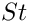does not need to be set, since it already defaults to a value of 1.0.

//============start_of_create_free_surface_elements======================
/// Create elements that impose the kinematic and dynamic bcs
/// for the pseudo-solid fluid mesh
//=======================================================================
template<class ELEMENT>
{
// Volume constraint element stores the Data item that stores
// volume conservation. Which value is the pressure stored in?
//Loop over the free surface boundaries
unsigned nb=Fluid_mesh_pt->nboundary();
for(unsigned b=First_bubble_boundary_id;b<nb;b++)
{
// How many bulk fluid elements are adjacent to boundary b?
unsigned n_element = Fluid_mesh_pt->nboundary_element(b);
// Loop over the bulk fluid elements adjacent to boundary b?
for(unsigned e=0;e<n_element;e++)
{
// Get pointer to the bulk fluid element that is
ELEMENT* bulk_elem_pt = dynamic_cast<ELEMENT*>(
Fluid_mesh_pt->boundary_element_pt(b,e));
//Find the index of the face of element e along boundary b
int face_index = Fluid_mesh_pt->face_index_at_boundary(b,e);
// Create new element
ElasticLineFluidInterfaceElement<ELEMENT>* el_pt =
new ElasticLineFluidInterfaceElement<ELEMENT>(
bulk_elem_pt,face_index);
// Add it to the mesh
el_pt->set_boundary_number_in_bulk_mesh(b);
//Specify the capillary number
el_pt->ca_pt() = &Problem_Parameter::Ca;
// Specify the bubble pressure (pointer to Data object and
// index of value within that Data object that corresponds
el_pt->set_external_pressure_data(
}
}
}
// end of create_free_surface_elements
void create_free_surface_elements()
Create free surface elements.

The volume constraint elements are created in a similar way. Recall that the LineVolumeConstraintBoundingSolidElements compute the line integrals in equation (10) while the VolumeConstraintElement addsto this equation.

//============start_of_create_volume_constraint_elements=================
/// Create elements that impose volume constraint on the bubble
//=======================================================================
template<class ELEMENT>
{
// Add volume constraint element to the mesh
//Loop over the free surface boundaries
unsigned nb=Fluid_mesh_pt->nboundary();
for(unsigned b=First_bubble_boundary_id;b<nb;b++)
{
// How many bulk fluid elements are adjacent to boundary b?
unsigned n_element = Fluid_mesh_pt->nboundary_element(b);
// Loop over the bulk fluid elements adjacent to boundary b?
for(unsigned e=0;e<n_element;e++)
{
// Get pointer to the bulk fluid element that is
ELEMENT* bulk_elem_pt = dynamic_cast<ELEMENT*>(
Fluid_mesh_pt->boundary_element_pt(b,e));
//Find the index of the face of element e along boundary b
int face_index = Fluid_mesh_pt->face_index_at_boundary(b,e);
// Create new element
ElasticLineVolumeConstraintBoundingElement<ELEMENT>* el_pt =
new ElasticLineVolumeConstraintBoundingElement<ELEMENT>(
bulk_elem_pt,face_index);
//Set the "master" volume constraint element
el_pt->set_volume_constraint_element(Vol_constraint_el_pt);
// Add it to the mesh
}
}
}
// end of create_volume_constraint_elements
void create_volume_constraint_elements()
Create elements that impose volume constraint on the bubble.

# Post-processing

This member function documents the computed solution after every Newton solve.

//==start_of_doc_solution=================================================
/// Doc the solution
//========================================================================
template<class ELEMENT>
void BubbleInChannelProblem<ELEMENT>::doc_solution(const std::string& comment)
{
oomph_info << "Docing step: " << Problem_Parameter::Doc_info.number()
<< std::endl;
ofstream some_file;
char filename;
sprintf(filename,"%s/soln%i.dat",
Problem_Parameter::Doc_info.directory().c_str(),
// Number of plot points
unsigned npts;
npts=5;
// Compute errors and assign to each element for plotting
double max_err;
double min_err;
compute_error_estimate(max_err,min_err);
// Assemble square of L2 norm
double square_of_l2_norm=0.0;
unsigned nel=Fluid_mesh_pt->nelement();
for (unsigned e=0;e<nel;e++)
{
square_of_l2_norm+=
dynamic_cast<ELEMENT*>(this->Fluid_mesh_pt->element_pt(e))->
square_of_l2_norm();
}
Problem_Parameter::Norm_file << sqrt(square_of_l2_norm) << std::endl;
some_file.open(filename);
some_file << dynamic_cast<ELEMENT*>(this->Fluid_mesh_pt->element_pt(0))
->variable_identifier();
this->Fluid_mesh_pt->output(some_file,npts);
some_file << "TEXT X = 25, Y = 78, CS=FRAME T = \"Global Step "
<< Problem_Parameter::Doc_info.number() << " "
<< comment << "\"\n";
some_file.close();
// Output boundaries
sprintf(filename,"%s/boundaries%i.dat",
Problem_Parameter::Doc_info.directory().c_str(),
some_file.open(filename);
this->Fluid_mesh_pt->output_boundaries(some_file);
some_file.close();
// Get max/min area
double max_area;
double min_area;
Fluid_mesh_pt->max_and_min_element_size(max_area, min_area);
// Get total volume enclosed by face elements (ignore first one)
double vol=0.0;
// Write trace file
<< this->time_pt()->time() << " "
<< Fluid_mesh_pt->nelement() << " "
<< max_area << " "
<< min_area << " "
<< max_err << " "
<< min_err << " "
<< sqrt(square_of_l2_norm) << " "
<< vol << " "
<< std::endl;
// Increment the doc_info number
} //end_of_doc_solution
void doc_solution(const std::string &comment="")
Doc the solution.

## Mesh adaptation for problems with 'closed' free boundaries

We described in another tutorial how oomph-lib employs a two-stage process for the (re-)generation of unstructured meshes in domains whose curvilinear boundaries are represented by GeomObjects: we initially sample the GeomObject at a user-specified number of points (equally spaced along the relevant section of the GeomObject) to create the vertices for an initial polygonal representation of the curvilinear boundary. This polygonal boundary representation is used to generate a new mesh with Triangle. The nodes on the domain boundaries are then "snapped" onto the curvilinear boundaries where required.

In principle, the same methodology can be (and is) employed for the mesh regeneration in free-surface problems. However, in a free-surface problem the curvilinear boundary evolves freely as part of the solution and is therefore not described by a user-specified GeomObject. When re-generating the mesh we therefore create a temporary GeomObject by attaching FaceElements to the relevant mesh boundaries of the existing mesh. We then use the vertices of the face elements to create a polyline representation of the boundary. This is illustrated in the figure below which shows part of the original mesh (the nodes and triangular elements adjacent to the boundary) in black. The blue lines represent the FaceElements that are erected on the current curvilinear domain boundary (as defined by the boundaries of the "bulk" elements). The vertices of these FaceElements (red hollow circles) provide the vertices for the polyline representation of the boundary (red line).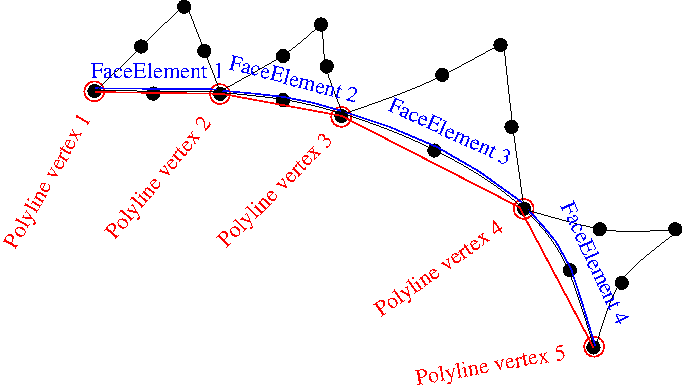Sketch illustrating the generation of a polyline along the curvilinear domain boundary.

Using this polyline representation of the boundary, a new mesh is built using Triangle. Depending on the target areas specified by the spatial error estimator, Triangle may erect multiple elements along each polyline segment. For instance in the figure below three elements have been created along the polyline segment created from FaceElement 3 in the original mesh.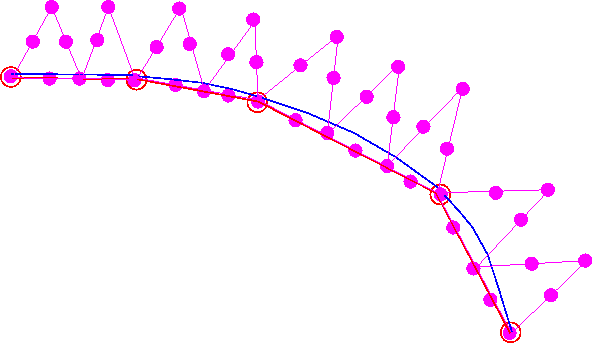Sketch showing the new mesh generated by Triangle, using (i) the polygonal representation of the boundary and (ii) the area targets provided by the spatial error estimator.

Next, the boundary coordinates are set up and the nodes are snapped onto the curvilinear boundary that is still defined by the FaceElements that were attached to the original mesh, as shown below.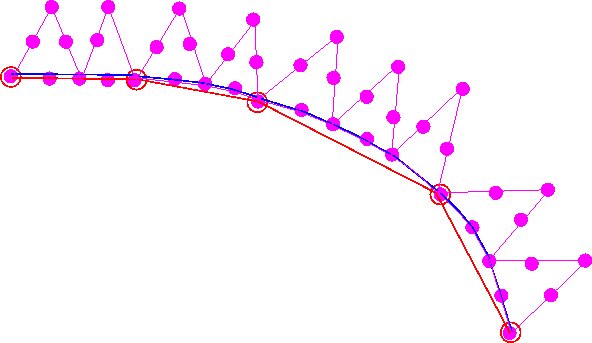Sketch showing the mesh following the snapping of nodes to the curvilinear boundary.

 IMPORTANT: Note that the initial shape of moving free boundaries must not be described by TriangleMeshCurviLines. While the use of TriangleMeshCurviLines would ensure that the nodes are initially located exactly on the curvilinear boundary described by the associated GeomObject without having to "snap" them to their desired position, a problem arises when the mesh is adapted. When this happens, nodes on curvilinear boundaries that are described by TriangleMeshCurviLines are placed on the (presumably unchanged) geometry defined by the associated GeomObject rather than being placed on the deformed boundary as described above – the free surface therefore keeps jumping back to its initial position whenever the mesh is adapted which is unlikely to be desired!

## Modifications to the basic mesh re-generation procedure

The procedure described above is very robust and works satisfactorily in the example problem discussed in this tutorial. Below we discuss a a few optional modifications to the mesh regeneration procedure that are helpful to deal with complications that can arise in certain circumstances:

### Unrefinement of polylines

As discussed above, Triangle generates a new mesh, based on (i) the polygonal representation of the boundary and (ii) the area targets provided by the spatial error estimator for the bulk elements. The polygonal boundary representation defines the minimum number of bulk elements that are generated next to the boundary – we showed above how multiple elements can be erected on a given boundary segment. However, since Triangle never merges any boundary segments this procedure can result in unnecessarily fine meshes near the boundary: once the bulk mesh has been refined to a certain level, the polygonal boundary representation cannot be coarsened, even if the spatial error estimator would allow much larger elements to be created at a later stage of the simulation.

We therefore provide the option to coarsen the polygonal boundary representation following its creation from the FaceElements that are attached to the current mesh. This is done by assessing if the (geometrical) boundary representation is unnecessarily fine, judged by how close any three adjacent vertices are to a straight line. This is illustrated in the sketch below: To assess if the middle vertex can be deleted, we determine the height of a circular segment connecting the three vertices. If the ratio of this height,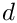, to the distance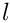between the two outer vertices is less than a user-defined tolerance (i.e. if the local curvature is so small that the middle vertex is not required to represent the boundary sufficiently accurately), the middle vertex is deleted.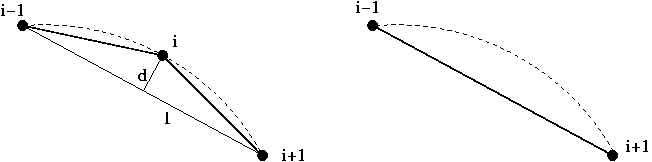Sketch illustrating the criterion for the unrefinement of polylines.

The tolerance for the unrefinement of polygons is set by the function

TriangleMesh::set_polyline_unrefinement_tolerance(...)

By default we allow polyline unrefinement (with a default tolerance of 0.04). Polyline unrefinement can be disabled by calling

TriangleMesh::disable_polyline_unrefinement()

where TriangleMesh is a base for the TriangleMeshClosedCurve class.

### Refinement of polylines

It is also possible that the free surface deforms in such a way that the polyline representation of the domain boundary becomes too inaccurate, e.g. because the representation of the solution only requires fairly large elements. Even though large elements may be sufficient to represent the solution, the mesh-regeneration tends to fail when "snapping" the nodes onto the highly-curved curvilinear boundary (typically because elements near the boundary become highly distorted or even inverted).

We therefore provide the option to refine the polygonal representation of the boundary employing a criterion similar to the one used for unrefinement discussed above. To assess the need for a boundary refinement we consider each boundary segment and compute the distance from segment's mid-point to its counterpart on the curvilinear boundary. If the ratio of the distance between these points,, to the length of the segment connecting the vertices,, is larger than a user-specifiable tolerance, the point on the curvilinear boundary becomes an additional vertex of the polyline and the original segment is split into two as illustrated in the figure below: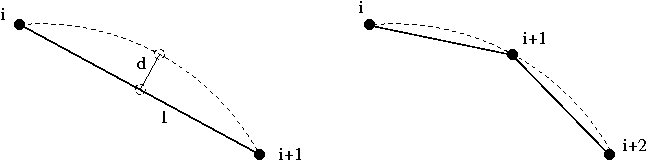Sketch illustrating the criterion for the refinement of polylines.

The tolerance for the refinement of polygons is set by the function

TriangleMesh::set_polyline_refinement_tolerance(...)

By default we allow polyline refinement (with a default tolerance of 0.08). Polyline refinement can be disabled by calling

TriangleMesh::disable_polyline_refinement()

### Redistribution of segments between polylines

The kinematic boundary condition (5) determines only the normal displacement of the boundary, hence the tangential displacement of nodes on the boundary is not controlled directly. It is therefore possible that nodes move along the perimeter of the curvilinear boundary and, as a result, one polyline may become much shorter than the others, as illustrated in the transition from a) to b) in the figure below. This is clearly undesirable and can be avoided by redistributing the vertices/segment between the polygon's constituent polylines such that each polyline spans an approximately equal fraction of the polygon's overall perimeter. This process is illustrated in b) and c) in the figure below. Note that the redistribution of segments does not change the shape of the polygonal boundary but merely the way in which it is represented in terms of polylines.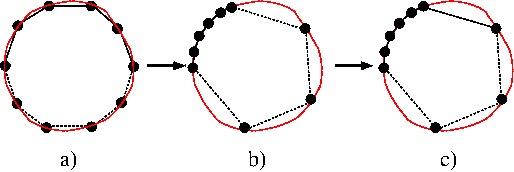Sketch illustrating the optional redistribution of polyline segments.

We provide the option to perform this step immediately after the generation of the updated polyline representation for the curvilinear boundary (and before the boundary refinement/unrefinement discussed above). Given that each polyline represents a distinct mesh boundary, the redistribution of segments between different polylines moves nodes from one boundary to another and, in general, this is clearly undesirable. Therefore, the redistribution of segments is is deactivated by default and must be activated by calling the function

TriangleMeshPolygon::enable_redistribution_of_segments_between_polylines()

Note that the redistribution of segments is not possible/sensible for TriangleMeshClosedCurve formed by TriangleMeshCurviLines since such boundaries are associated with a specific, continuous GeomObject with specific start/end coordinates.

## Exercises

1. As discussed above, we start the simulation by performing an initial steady Newton solve during which we deform the polygonal boundary that represents the air-liquid interface into its static equilibrium shape – a circle enclosing the required volume. This may seem like a rather costly way of creating a circular interface. Why not simply move the nodes on that boundary manually to their "correct" positions by adjusting their radial positions after returning from the mesh constructor? To explore this question, snap the required nodes manually onto the circular boundary and call the steady Newton solver. Why is the initial residual not equal to zero, even though we have manually assigned the correct solution as an initial guess?
2. Comment out create_volume_constraint_elements() and delete_volume_constraint_elements() throughout the code and explain what happens.
3. Experiment with the refinement/unrefinement of polylines and explore the option to re-distribute segments between the polylines that define the boundaries of the bubble. Specifically, change the initial polygonal representation the bubble surface such that the first TriangleMeshPolyLine` only represents 1/4 of the perimeter while the second one represents the remaining 3/4. Confirm that, following the mesh adaptation, the bubble boundaries are adjusted such that each boundary occupies approximately 1/2 of the bubble surface when the redistribution of polylines is enabled.